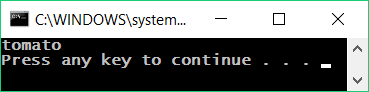Simple If Conditions

In programming we often check particular conditions and perform various actions depending on the result of the check. This is done by if condition, which has the following structure:

if (condition)
{
// condition body;
}

Video: Simple If / If-Else Conditions

Watch the video lesson about the simple if-conditions: https://youtu.be/M-G4nOaVDVA.

We read the grade from the console and check if it's excellent (≥ 5.50).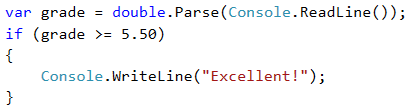Test the code from the example locally. Try entering different grades, for example 4.75, 5.49, 5.50 and 6.00. For grades less than 5.50, the program will not give any output, however if the grade is 5.50 or greater, the output would be "Excellent!".

Testing in the Judge System

Test your solution from the example here:
https://judge.softuni.org/Contests/Practice/Index/506#0.

If-Else Conditions

The if construction may also contain an else clause to give a specific action in case the Boolean expression (which is set at the beginning if (bool expression) ) returns a negative result (false). Built this way, the conditional statement is called if-else and its behavior is as follows: if the result of the condition is positive (true) – we perform some actions, when it is negative (false) – others. The format of the construction is:

if (condition)
{
// condition body;
}
else
{
// else construction body;
}

Like the example above, we read the grade from the console and check if it's excellent, but this time we should output the result in both cases.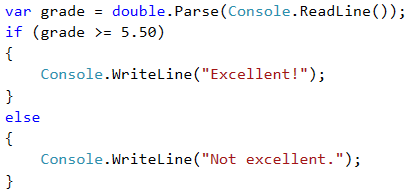Testing in Judge System

Test your solution from the example here: https://judge.softuni.org/Contests/Practice/Index/506#1.

About the Curly Braces {} After If / Else

When we have only one command in the body of the if construction, we can skip the curly brackets, indicating the conditional operator body. When we want to execute block of code (group of commands), curly brackets are required. In case we drop them, only the first line after the if clause will be executed.It's a good practice to always put curly braces, because it makes our code more readable and cleaner.

Here is an example where dropping curly braces leads to confusion: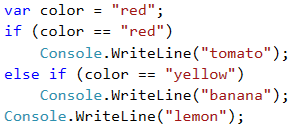Executing the above code will output the following console result: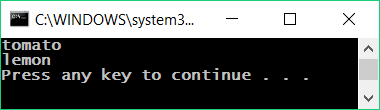With curly braces: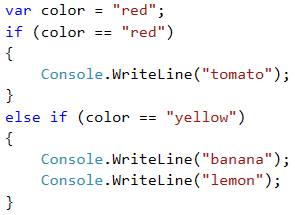The following output will be printed on the console: